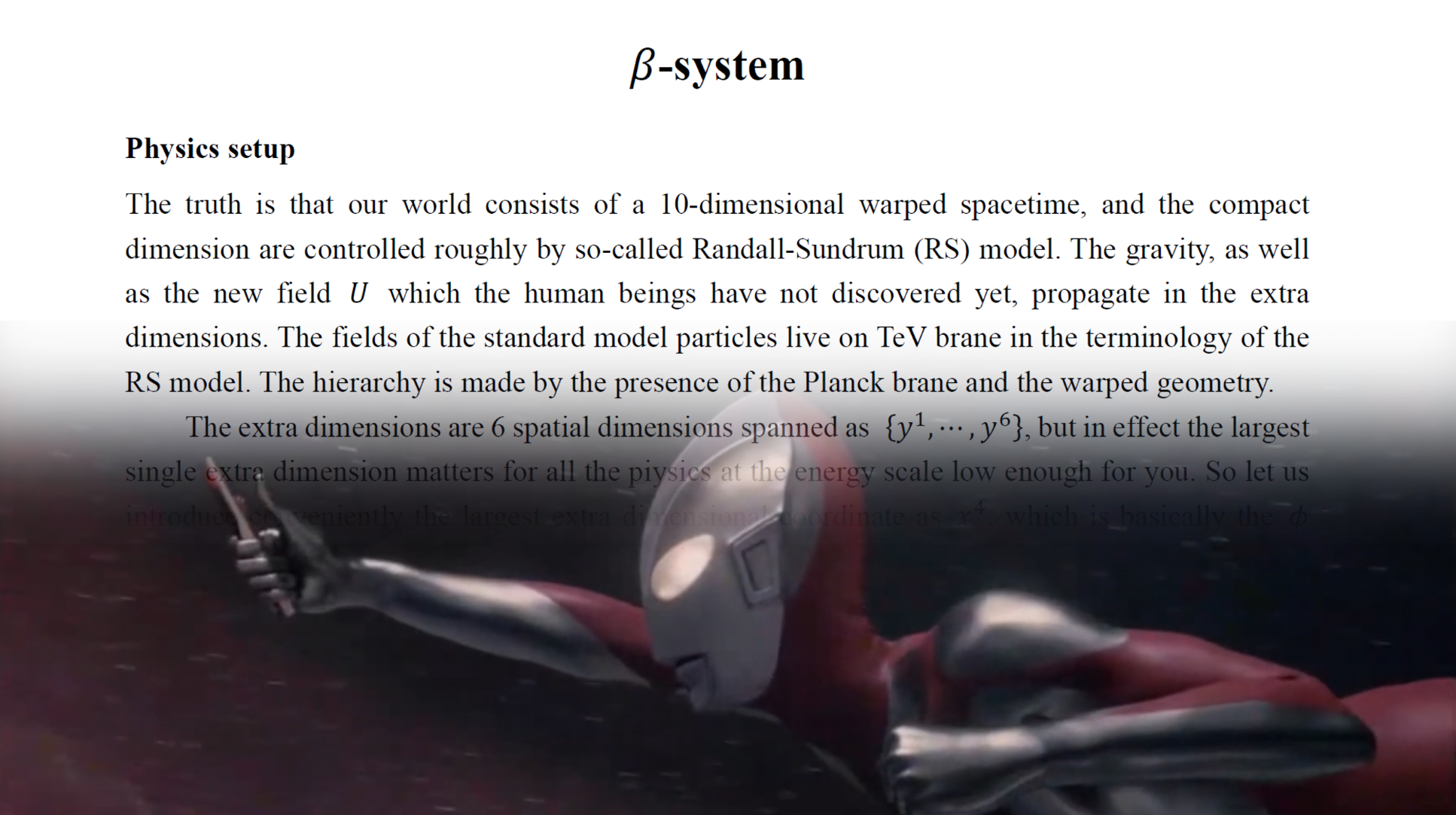# $\beta$-system#

## Physics setup#

The truth is that our world consists of a 10-dimensional warped spacetime, and the compact dimension are controlled roughly by so-called Randall-Sundrum (RS) model. The gravity, as well as the new field $U$ which the human beings have not discovered yet, propagate in the extra dimensions. The fields of the standard model particles live on TeV brane in the terminology of the RS model. The hierarchy is made by the presence of the Planck brane and the warped geometry.

The extra dimensions are 6 spatial dimensions spanned as ${y^1,\cdots,y^6}$, but in effect the largest single extra dimension matters for all the physics at the energy scale low enough for you. So let us introduce conveniently the largest extra dimensional coordinate as $x^4$, which is basically the $\phi$ coordinate in the RS model. Then at low energy the extra dimensional physics reduces to the 5-dimensional model, whose spacetime is spanned by ${x^0,\cdots,x^3,x^4}$. The spacetime metric depends affectively only on $x^4$.

As I described above, there are two branes parallel to each other, and located at $$\phi=0,~~\phi=\pi.$$

In the dimensionless coordinate $\phi ≡ x^4/L$, where $L$ is the length scale of the extra dimension. The field content at $\phi =0$ brane is the standard model fields (and the dark matter fields which will be no use for you). To be precise, the standard model fields are effectively localized. And the field content at $\phi =\pi$, our “world of light,” is again no use for you, so I will not describe them here. However, the important is the field content which propagates also in the extra dimensions, $$U(x^0,\cdots,x^3,\phi),~~G_{MN}(x^0,\cdots,x^3,\phi).$$

Here of course the metric field $G$ has the indices $M,N=0,1,2,3,4$.

## Action of the whole higher dimensional universe#

The most important paint of the 5-dimensional Lagrangian is that the undiscovered field $U$ has a non-minimal coupling to the gravity. It is similar to the Higgs inflation model. Therefore the gravitational effect of the field $U$ is strong at the TeV brane in particular.

The mass of the $U$ particle (or more precisely, the lowest Kalnza-Klein mode of the 5-dimensional field $U$) is at 13.8 TeV, whose observation will determine the relation between the 5-dimensional warp factor and the 5-dimensional mass $M$ of the field $U$.

The action consists first of the RS model Lagrangian,

$$s=\int d^5x \sqrt{-G}\left[\left(2M^3R-\Lambda\right) + \delta\left(\phi-\pi\right){\cal L}_{\rm SM}+\delta\left(\phi\right){\cal L}_{\rm PL}\right].$$

The additional field has the following form of the action,

$$\delta S=\int d^5x\sqrt{-G} \left[ G^{MN}D_MUD_N\overline U -M^2\left|U\right|^2 +\epsilon_0\left|U\right|^2R +g\left|U\right|^2\delta\left(\phi\right)H^\uparrow H +\overline g\left|U\right|^2\delta\left(\phi-\pi\right){\cal L}’_{\rm PL} \right],$$

which allows indeed the noni-minimal coupling between the 5-dimensional field $U$ and the gravity. $H$ is the Higgs field which, as in the RS model, is written to show the hierarchy structure. The field $U$ is the key field, which strongly couples to the 5-dimensional gravity. The coupling parameter $\epsilon_0$, once is measured, will now governs the physics of the extra dimensions.